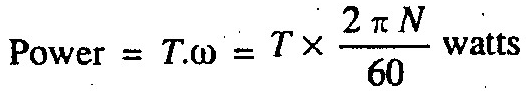# Mechanical Power | Definition | Formula

What is definition and formula of Mechanical Power?

It is the rate of doing work or workdone per unit time. In S.I. system of units, the unit of power is watt (briefly written as W) which is equal to 1N-m / s or 1J/s. Generally a bigger unit of power called kilowatt (briefly written as kW) is-used which is equal to 1000W.

Note: If T is the torque transmitted in N-m or J and ω is the angular speed in rad/s, then
Power = T.ωwhere    N = Speed in r.p.m.

Power in mechanical systems is the combination of forces and movement. In particular, power is the product of a force on an object and the object's velocity, or the product of a torque on a shaft and the shaft's angular velocity.

Mechanical power is also described as the time derivative of work. In mechanics, the work done by a force F on an object that travels along a curve C is given by the line integral:$Power in Mechanical Systems$